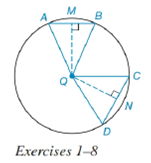Chapter 6.4, Problem 8EElementary Geometry For College St...

7th Edition
Alexander + 2 others
ISBN: 9781337614085

Solutions

Chapter
SectionElementary Geometry For College St...

7th Edition
Alexander + 2 others
ISBN: 9781337614085
Textbook Problem

In Exercises 1 to 8, use the figure provided.If Q N : Q M = 5 : 6 , write an inequality that compares m A B ⌢ to m C D ⌢ .To determine

To write:

An inequality that compares mAB to mCD.

Explanation

Given:

QN:QM=5:6 and the figure given below

Theorem:

In a circle (or in congruent circles) containing two unequal chords, the chord nearer the center of the circle has the greater length.

In a circle (or in congruent circles) containing two unequal chords, the longer chord corresponds to the greater minor arc.

Calculation:

Since, QN:QM=5:6, it means that QM¯<QN¯.

Using the theorem.

Since the chords are unequal and the distance of chords from center is also unequal i.e. QM¯<QN¯ and from the given figure.

The chord CD is at distance QN and the chord AB is at distance QM, we conclude that CD¯<AB¯

Still sussing out bartleby?

Check out a sample textbook solution.

See a sample solution

The Solution to Your Study Problems

Bartleby provides explanations to thousands of textbook problems written by our experts, many with advanced degrees!

Get Started

let f(x) = x3 + 5, g(x) = x2 2, and h(x) = 2x + 4. Find the rule for each function. 8. fgh

Applied Calculus for the Managerial, Life, and Social Sciences: A Brief Approach

If f is periodic, then f is periodic.

Single Variable Calculus: Early Transcendentals, Volume I

The slope of the tangent line to r = cos θ at is:

Study Guide for Stewart's Single Variable Calculus: Early Transcendentals, 8th